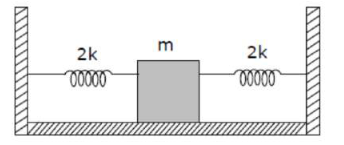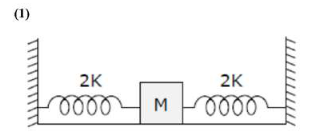# Two identical spring of spring constant '2K' are attached to a block of mass m and to fixed support (see figure).Question:

Two identical spring of spring constant '2K' are attached to a block of mass $m$ and to fixed support (see figure). When the mass is displaced from equilibrium position on either side, it executes simple harmonic motion. Then time period of oscillations of this system is:1. (1) $\pi \sqrt{\frac{m}{k}}$

2. (2) $\pi \sqrt{\frac{\mathrm{m}}{2 \mathrm{k}}}$

3. (3) $2 \pi \sqrt{\frac{m}{k}}$

4. (4) $2 \pi \sqrt{\frac{\mathrm{m}}{2 \mathrm{k}}}$

Correct Option: 1

Solution:Dut to parallel combination $\mathrm{K}_{\mathrm{eff}}=2 \mathrm{k}+2 \mathrm{k}$

$\because \mathrm{T}=2 \pi \sqrt{\frac{\mathrm{m}}{\mathrm{k}_{e f f}}}$

$=2 \pi \sqrt{\frac{m}{4 k}}$

$\mathrm{T}=\pi \sqrt{\frac{\mathrm{m}}{\mathrm{k}}}$